Most Affordable JEE | NEET | 8,9,10 Preparation by Kota's Top IITian Doctor Faculties

# NCERT Class 12 Maths Chapter 6 Exercise 6.2 Solutions - Application of Derivatives - Free PDF Download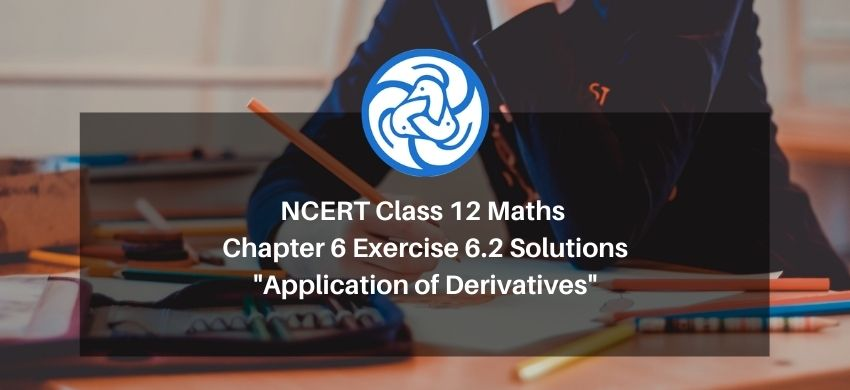Hey, are you a class 12 Student and Looking for Ways to Download Class 12 Maths Chapter 6 Exercise 6.2 Solutions? If Yes then you are at the right place.

All the solutions of Class 12 Math Chapter 6 exercise 6.2 is prepared by Kota’s top IITian’s Faculties by keeping Simplicity in mind.

If you want to score high in your class 12 Maths Exam then it is very important for you to have a good knowledge of all the important topics, so to learn and practice those topics you can use eSaral NCERT Solutions.

So, without wasting more time Let’s start.

### Download The PDF of NCERT Class 12 Math Chapter 6 Exercise 6.2 Solutions "Application of Derivatives"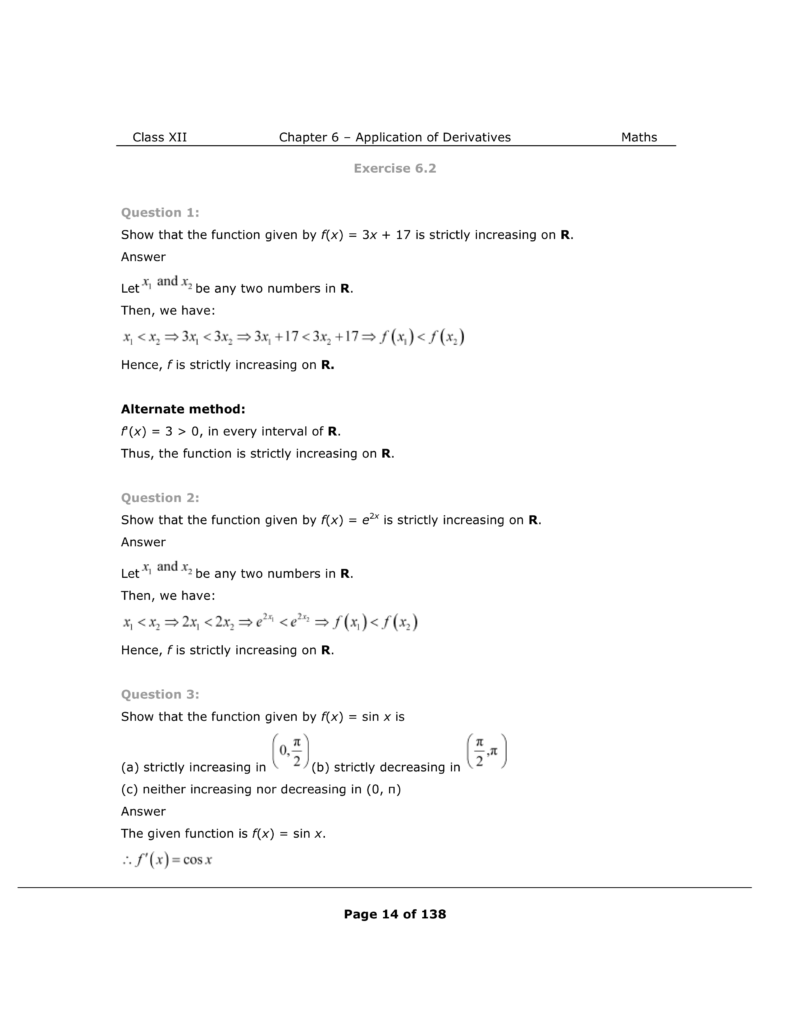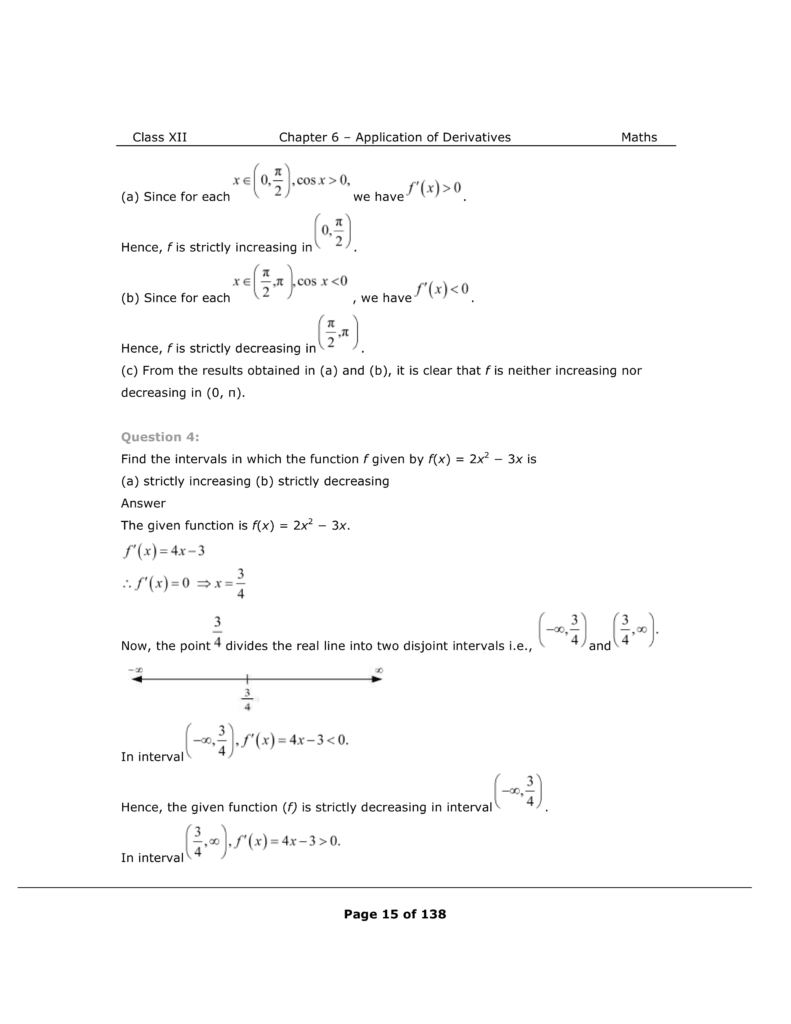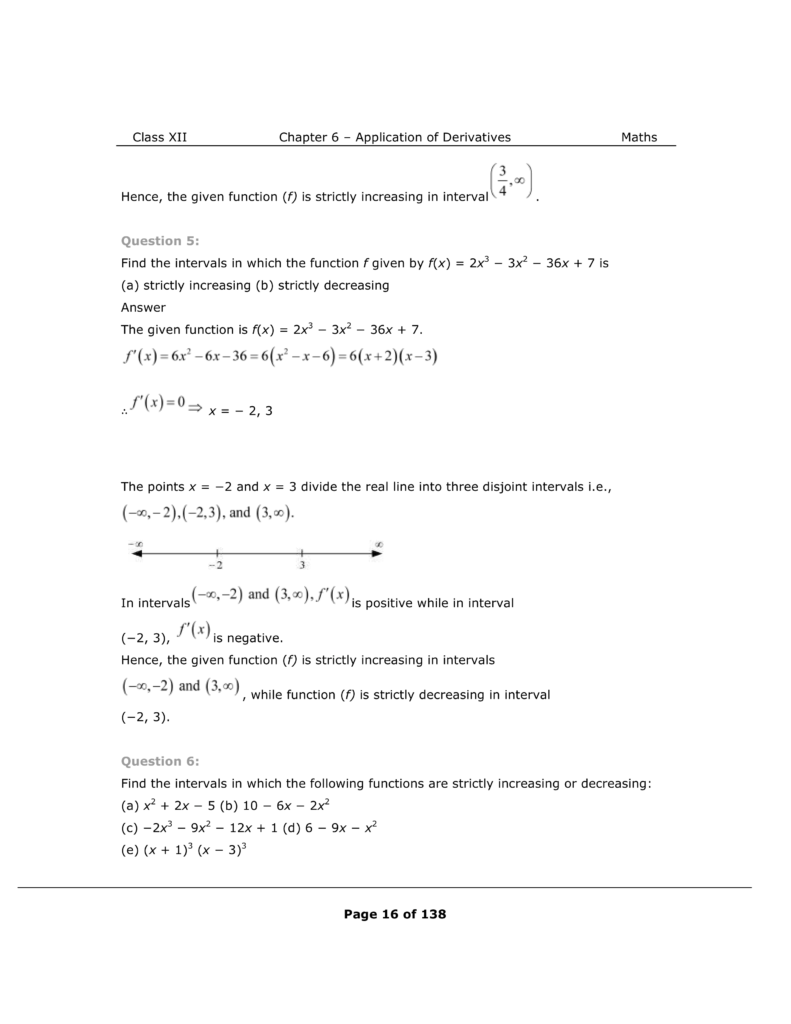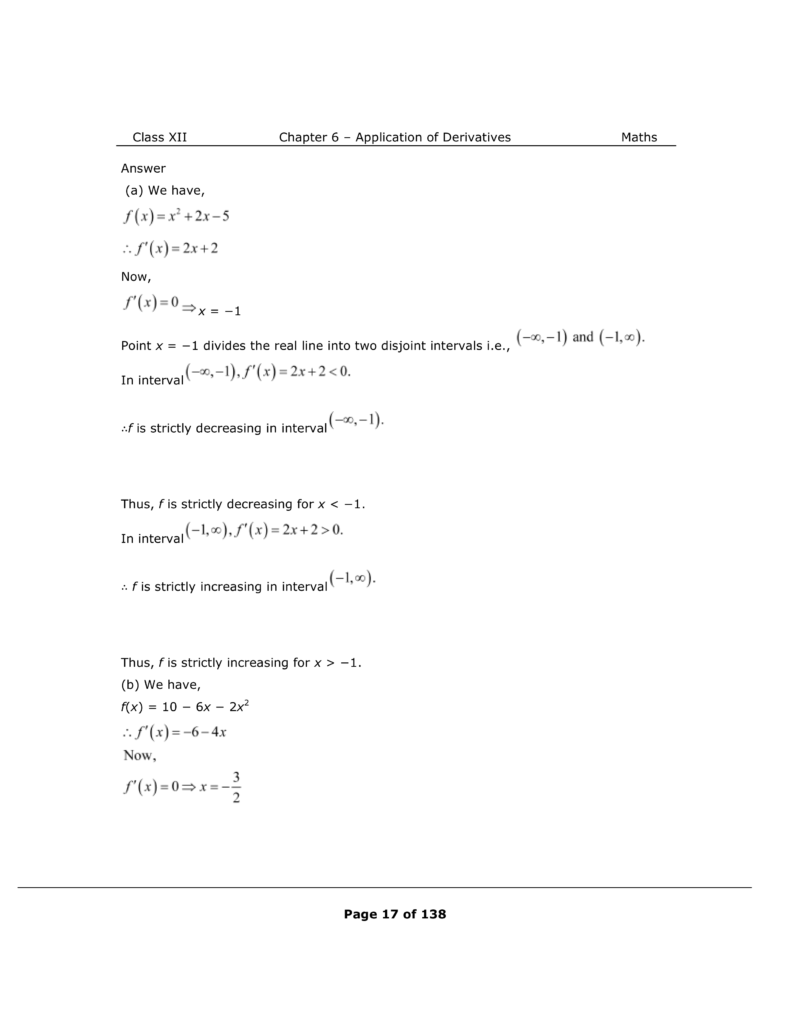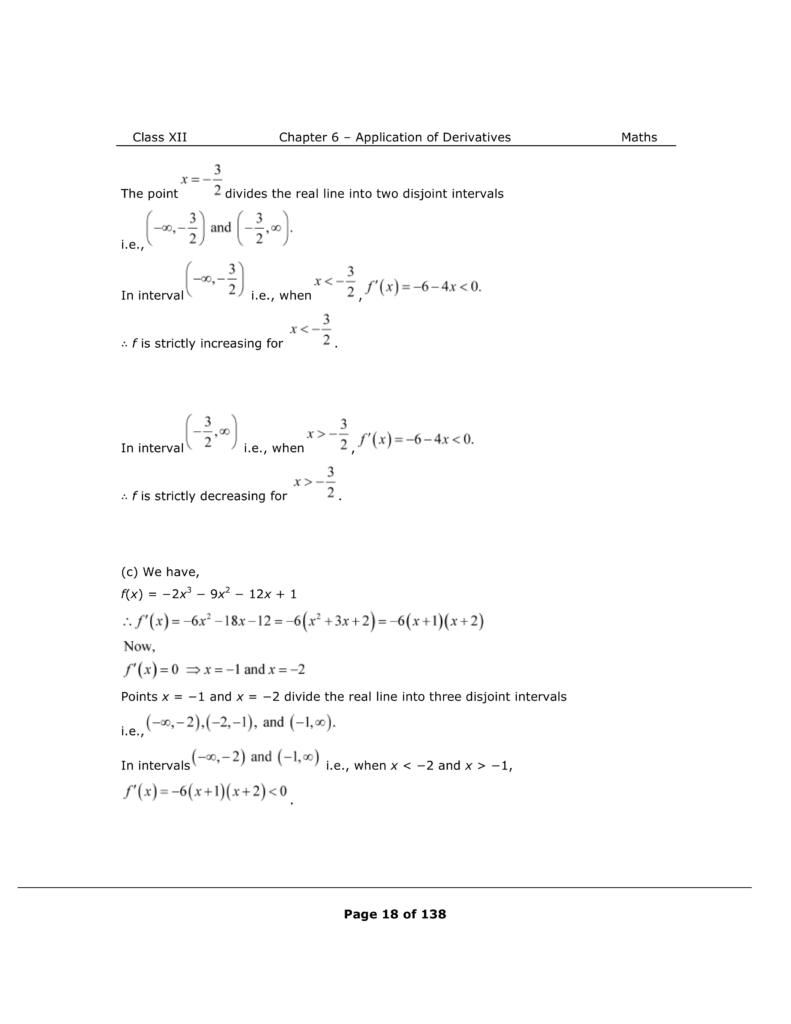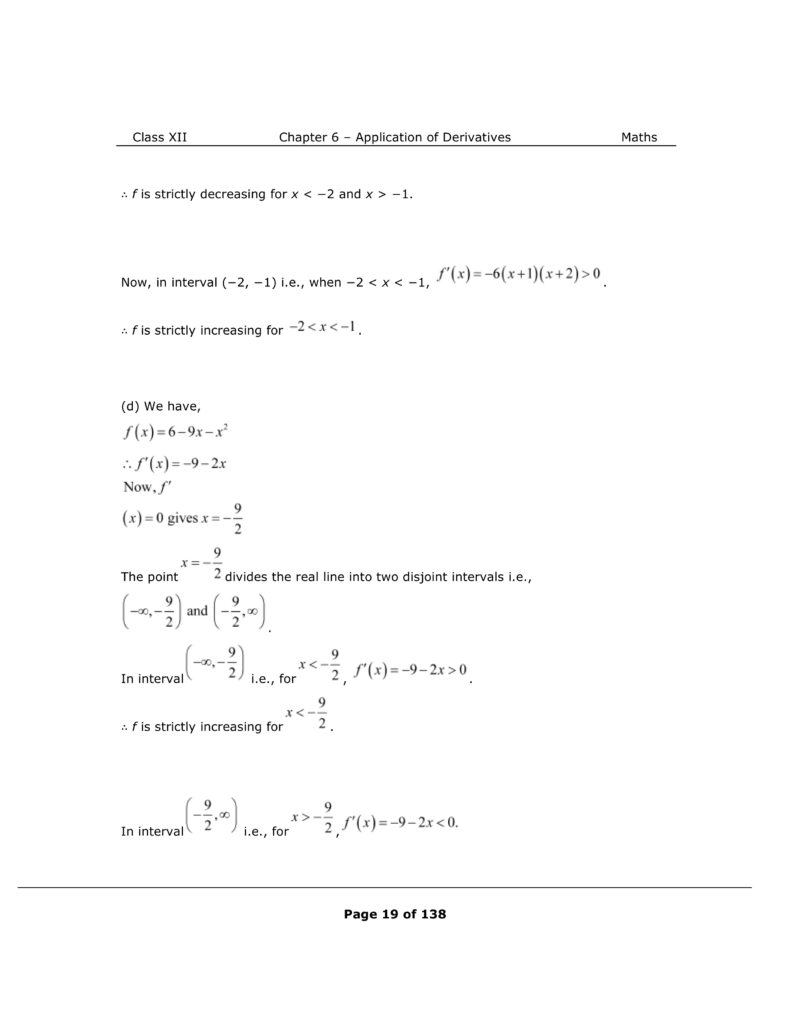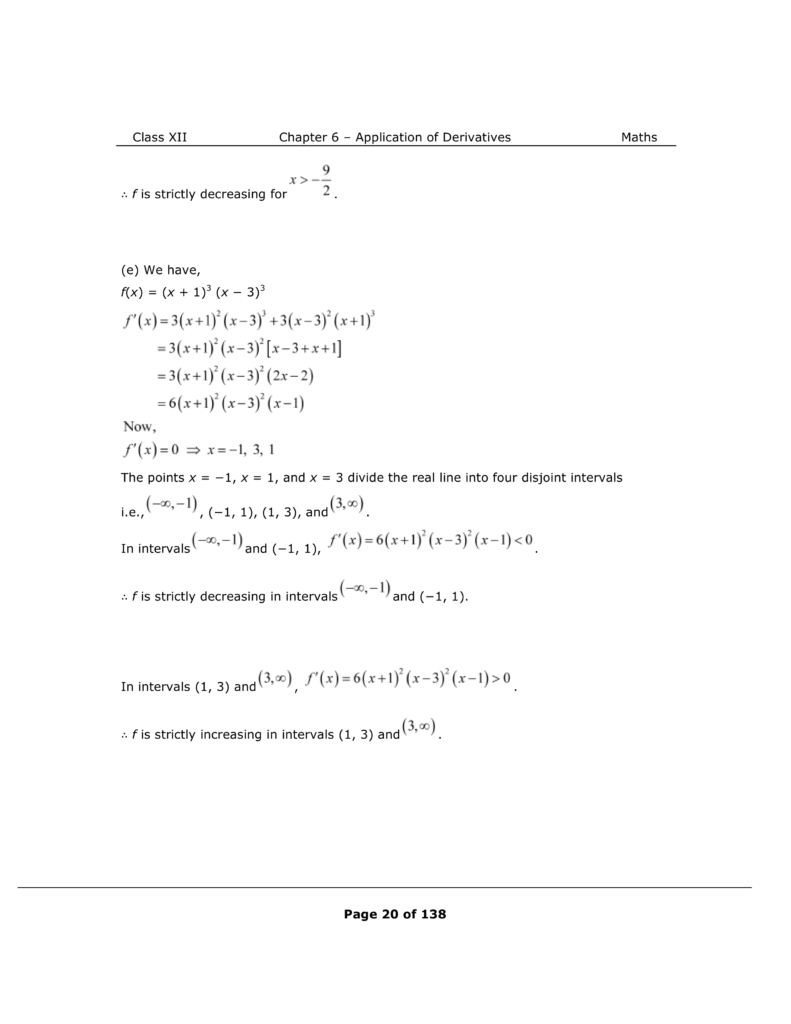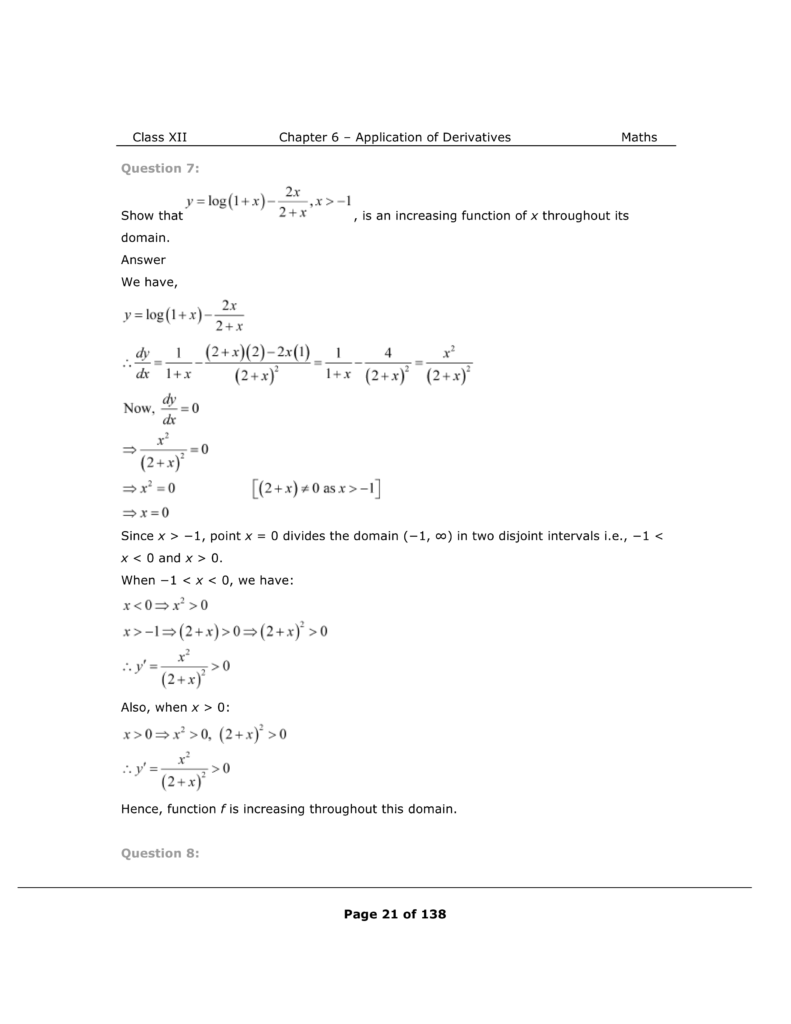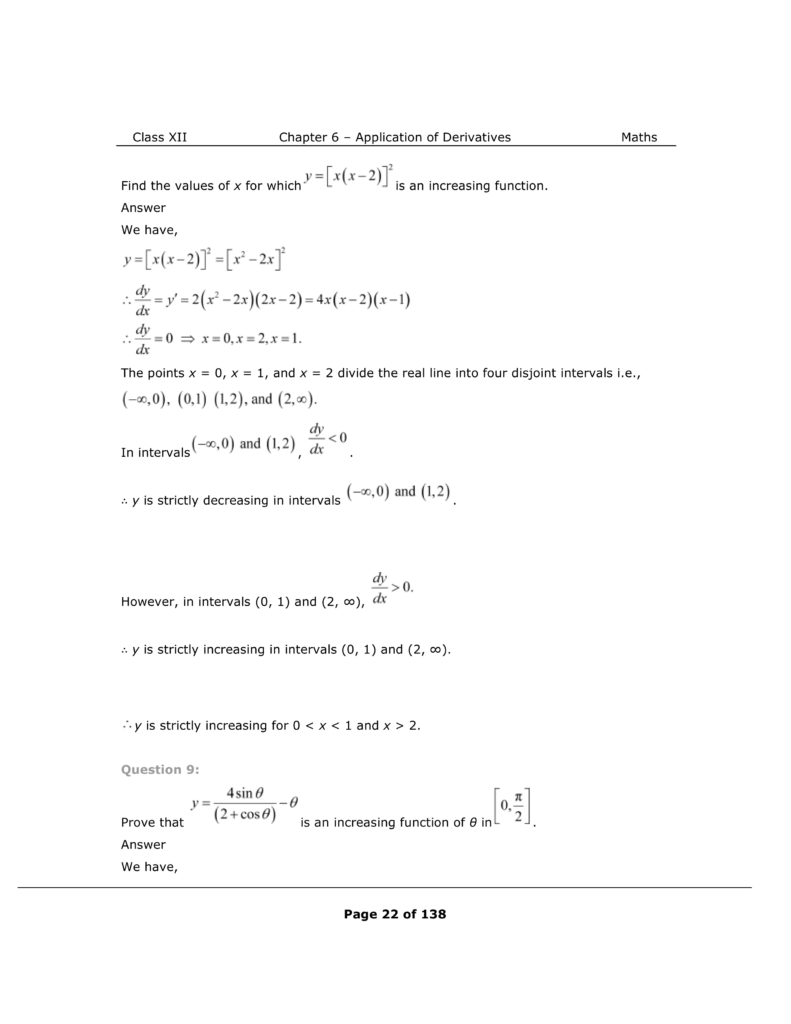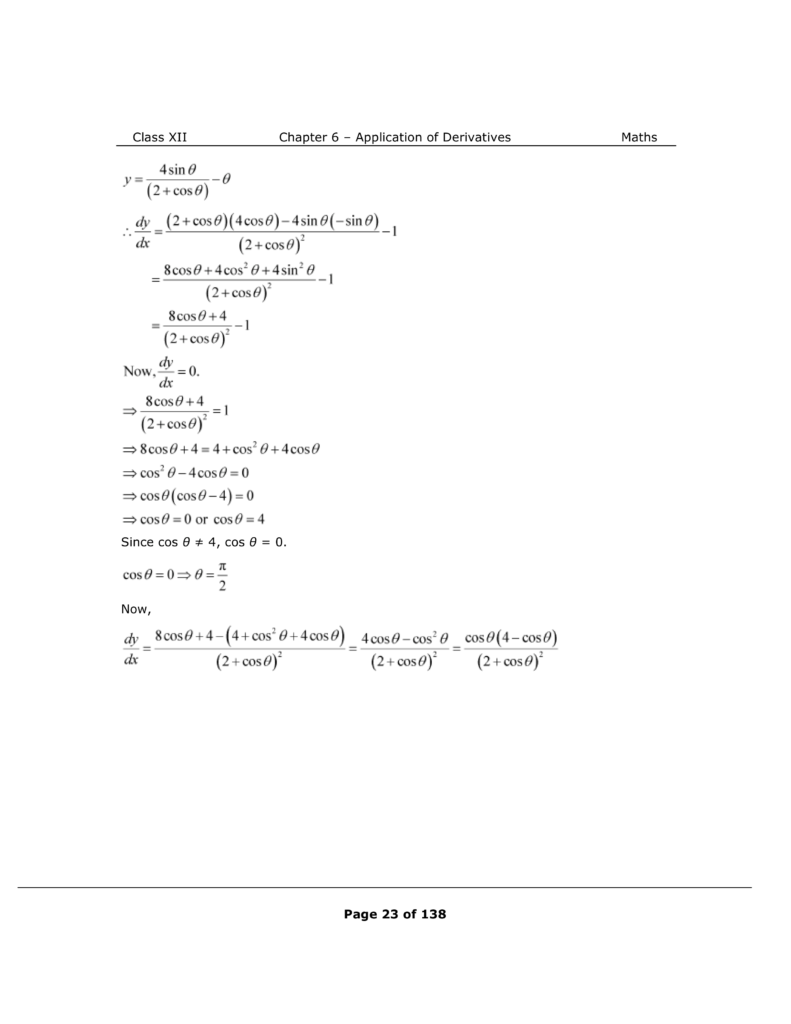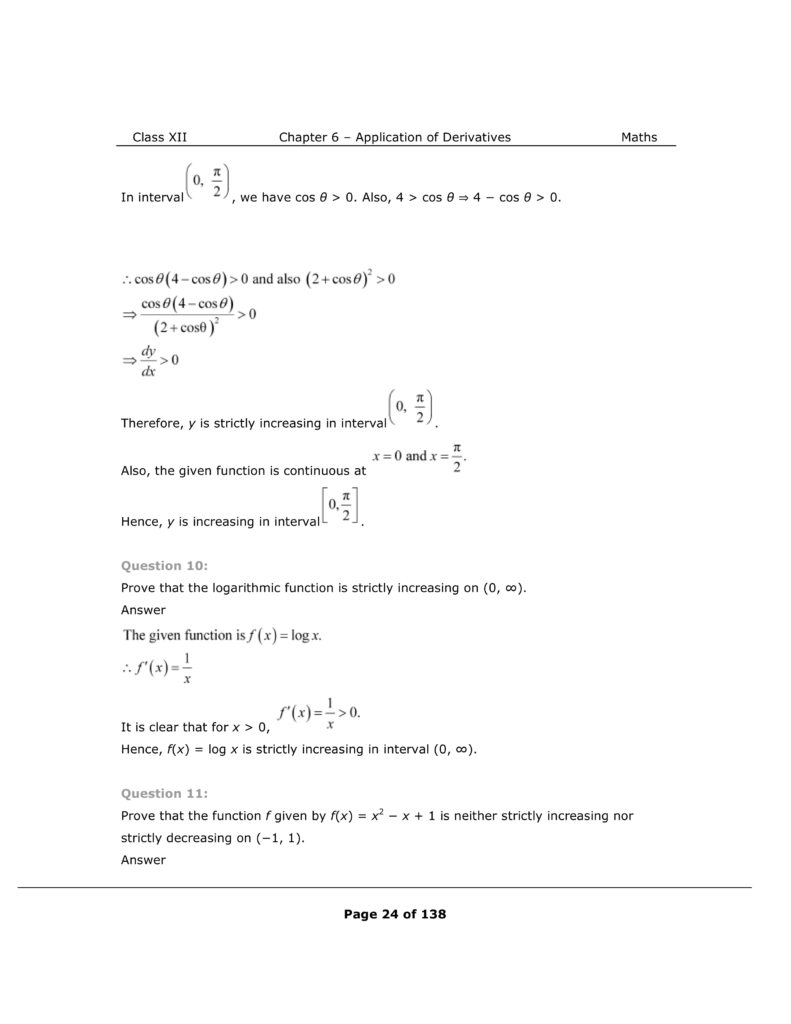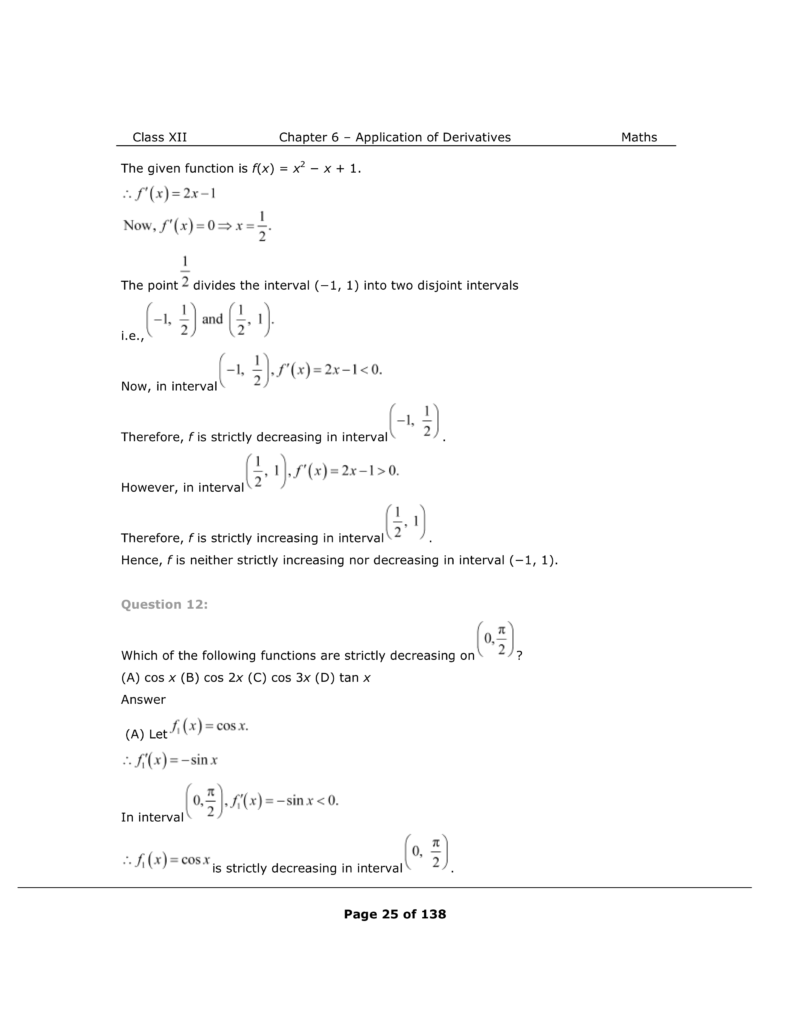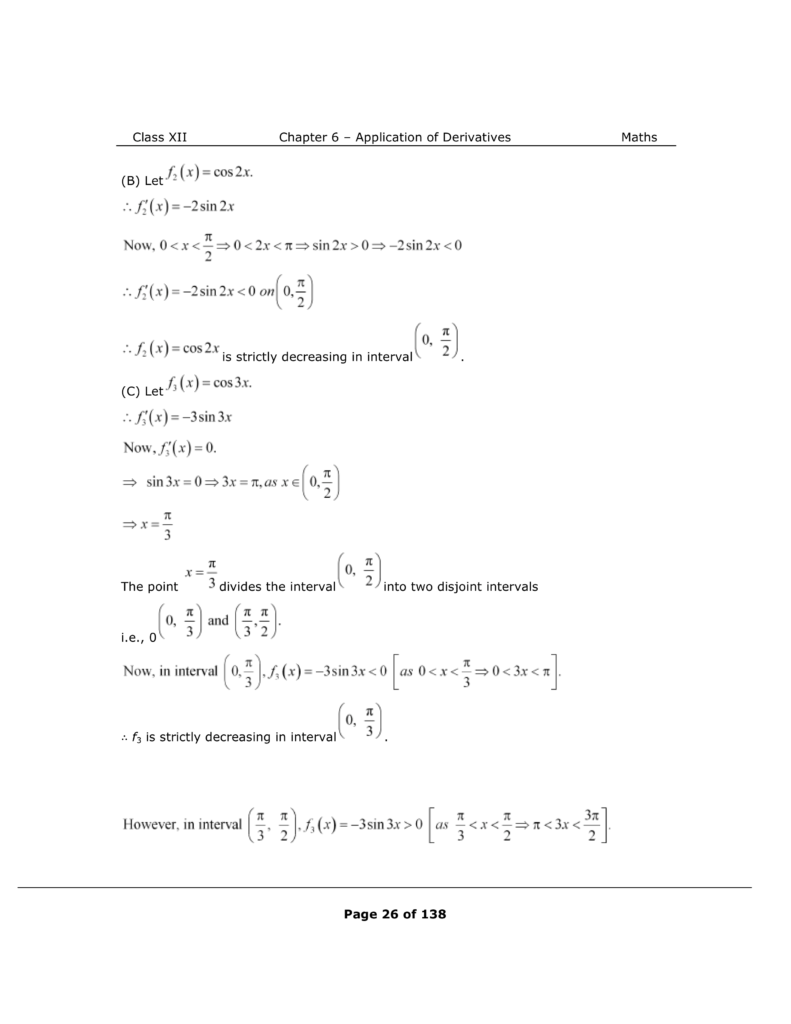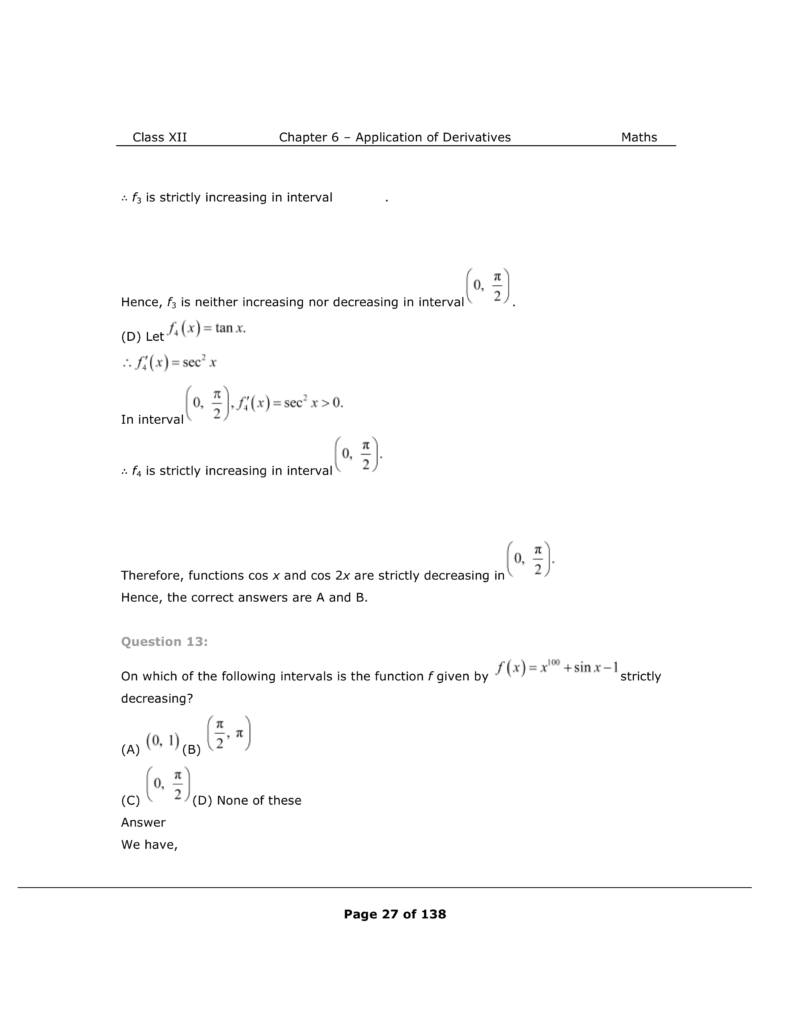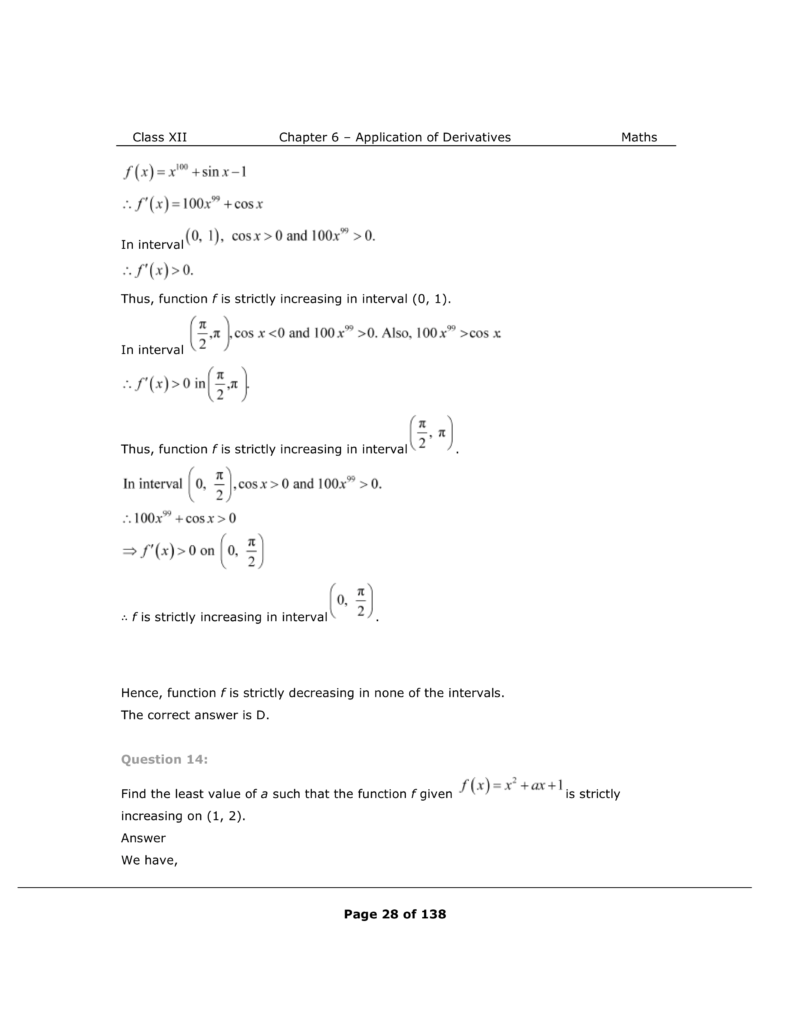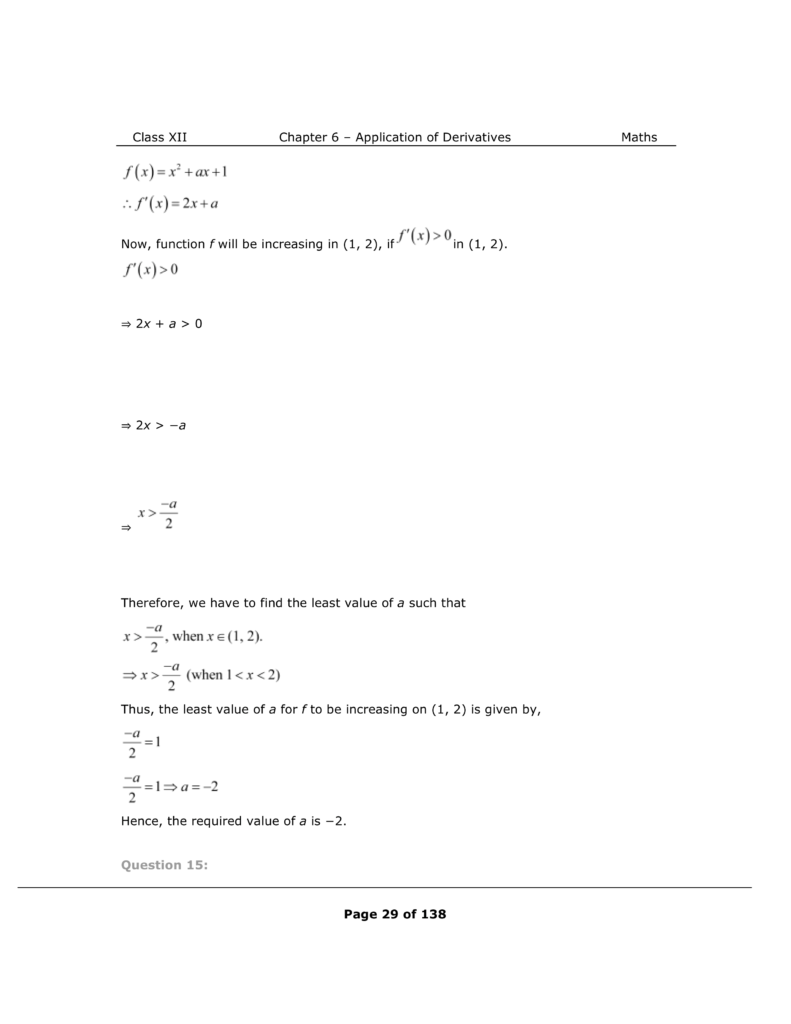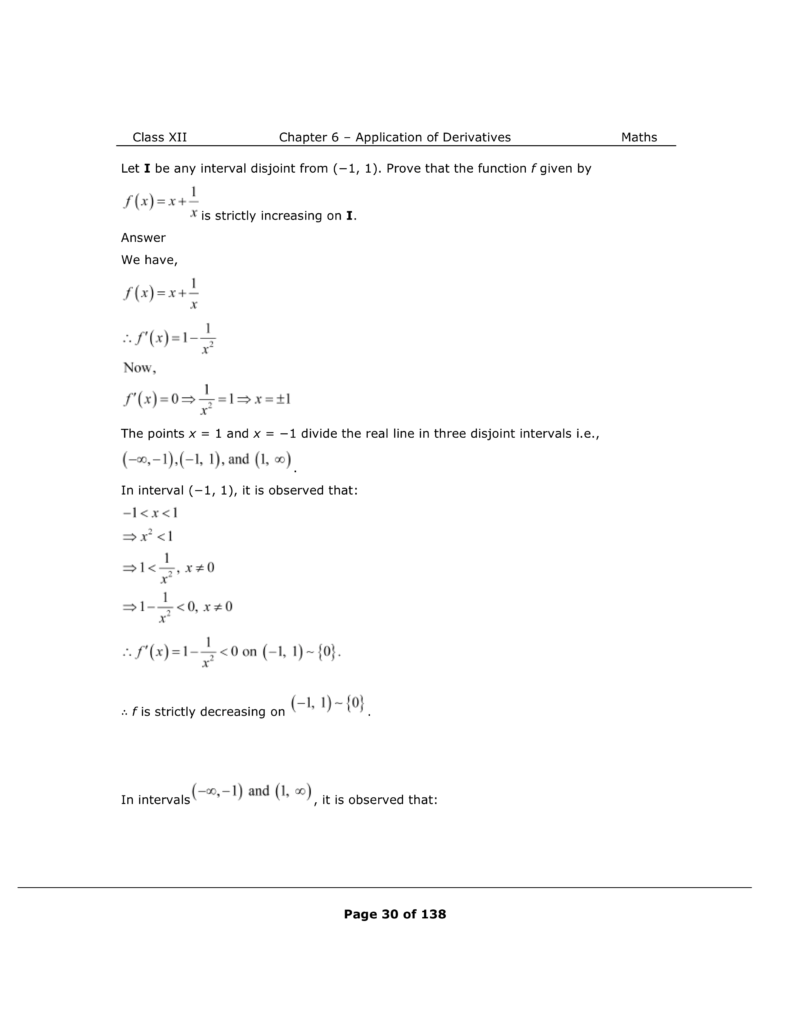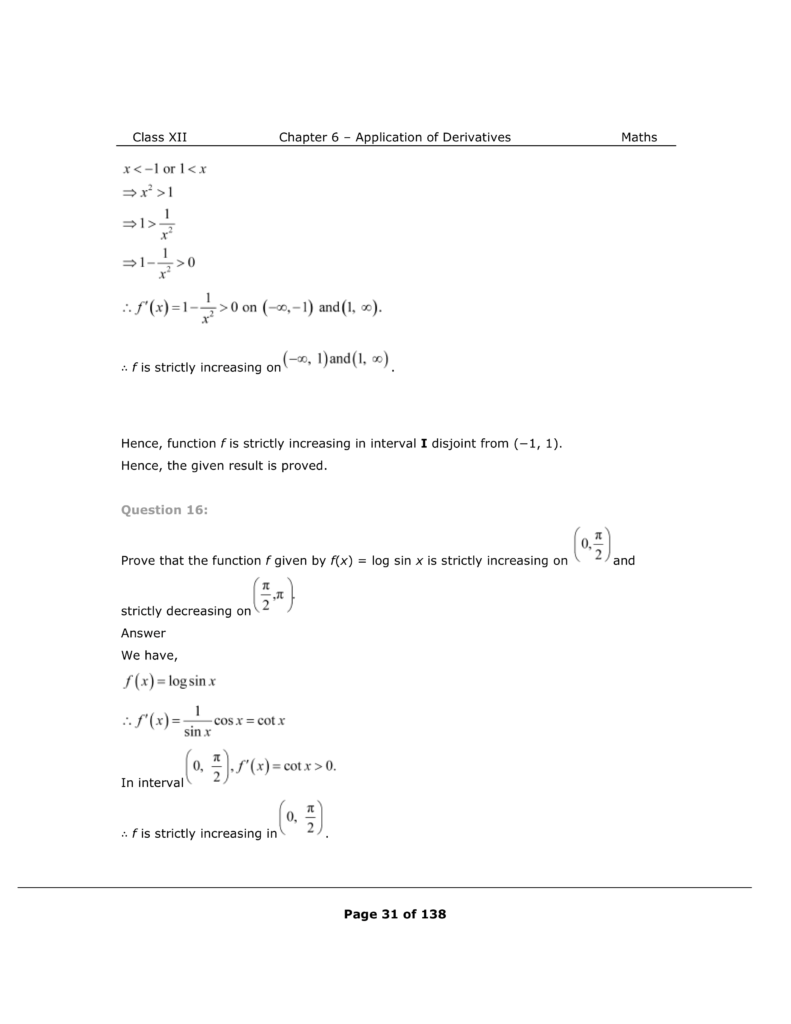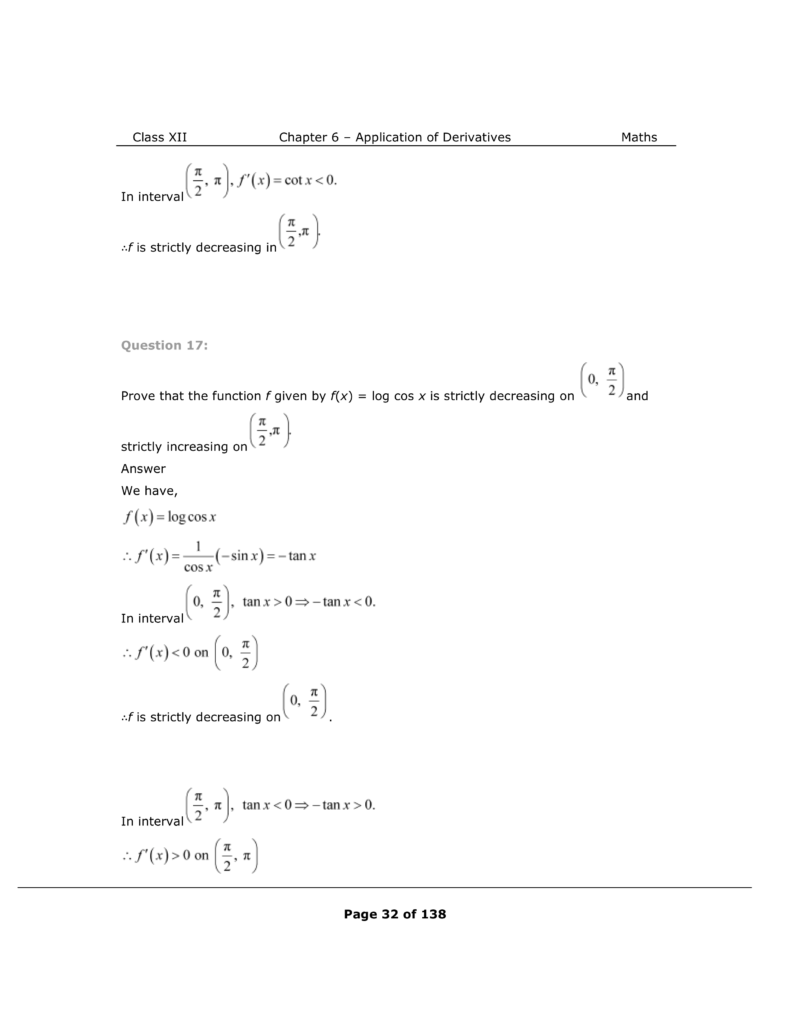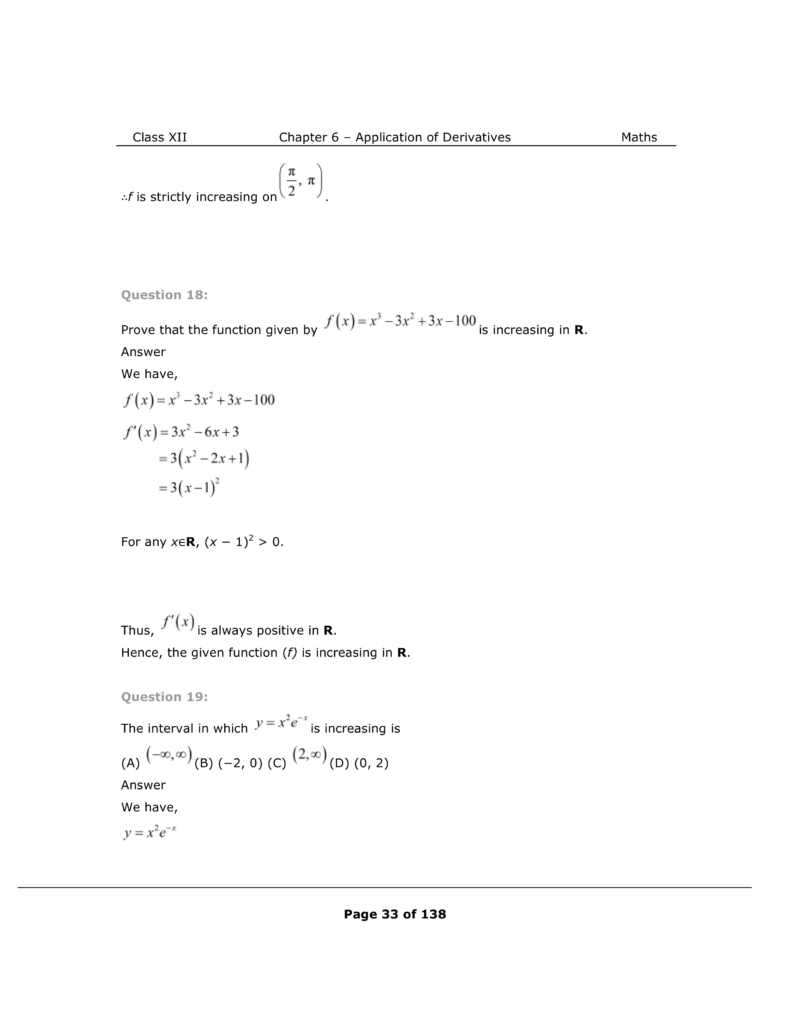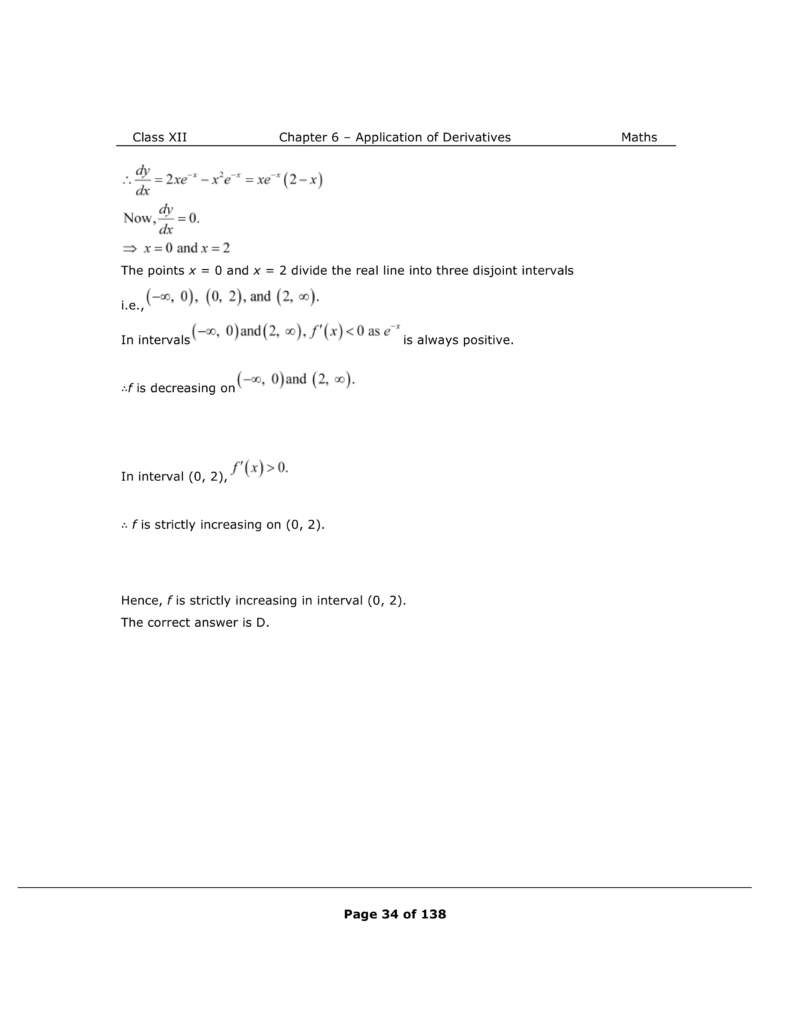NCERT Class 12 Math Book Free

NCERT Class 12 Maths Exemplar Free

Complete Solutions for Class 12 Maths chapter 6 Free

Class 12 maths chapter 5 exercise 5.1 Solutions Free

Class 12 maths chapter 5 exercise 5.2 Solutions Free

Class 12 maths chapter 5 exercise 5.3 Solutions Free

Class 12 maths chapter 5 exercise 5.4 Solutions Free

Class 12 maths chapter 5 exercise 5.5 Solutions Free

Class 12 maths chapter 5 exercise 5.6 Solutions Free

Class 12 maths chapter 5 exercise 5.7 Solutions Free

Class 12 maths chapter 5 exercise 5.8 Solutions Free

Class 12 maths chapter 5 Miscellaneous exercise Solutions Free

If you have any Confusion related to Class 12 Maths Chapter 6 Exercise 6.2 Solutions then feel free to ask in the comments section down below.

To watch Free Learning Videos on Class 12 by Kota’s top Faculties Install the eSaral App